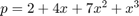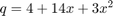# Derivatives with Matrices

Using simple matrix multiplication, we are able to find the derivative of, at least, polynomials. Our aim is to find the derivative of```% The derivative matrix, D, for polynomials of degree 3.
D = [0 1 0 0; 0 0 2 0; 0 0 0 3]
```
```D =

0     1     0     0
0     0     2     0
0     0     0     3

```
```% The polynomial, p,
p = [ 2; 4; 7; 1];

% The derivative of p (d/dx) is multiplication of matrices D and p

q = D*p

% The result is
```
```q =

4
14
3

```